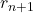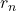## Consider the spreading of a rumor through a company of 1000 employees, allworking in the same building.We assume that the spreading of a rum

Question

Consider the spreading of a rumor through a company of 1000 employees, allworking in the same building.We assume that the spreading of a rumor is similar to the spreading of a contagious disease (see Example 3, Section 1.2) in that the number of people hearing the rumor each day is proportional to the product of the number who have heard the rumor previously and the number who have not heard the rumor. This is given by
rn+1 = rn + 1000krn(1000 ? rn)
where k is a parameter that depends on how fast the rumor spreads and n is the number of days. Assume k = 0.001 and further assume that four people initially have heard rumor. How soon will all 1000 employees have heard the rumor?

in progress 0
2 months 2021-07-23T12:13:46+00:00 1 Answers 0 views 0

On the 12th day, the rumor would have has to all the 1000 employees

Step-by-step explanation:

Given the data in the question;

so, lets consider the rumor spreading thorough the company of 1000 employee;

the given model is;=+ 1000k( 1000 –)

where k is the parameter that depends on how fast the rumor spreads, n is the number of days.

now, lets assume k = 0.0001, lets also assume r₀ = 4

so to find how soon all 1000 employees  will have heard the rumor  ;

let n be;

then r1 will be;

r1 = r₀ + ( 0.001)r₀(1000-r₀ )

so

r1 = 4 + ( 0.001) × 4× (1000 – 4 )

r1 = 7.984

r2 will be;

r2 = r1 + ( 0.001)r1(1000-r1 )

r2 =  7.984 + ( 0.001) × 7.984 × (1000 – 7.984 )

r2 = 15.904255744

r3  will be;

r3 = r2 + ( 0.001)r2(1000-r2 )

r3 = 15.904255744 + ( 0.001) × 15.904255744 × (1000 – 15.904255744 )

r3 = 31.555566137

Using the same formula and procedure by substituting n = 1,2,3,4,5,6,7,8,9,10,11,12.

we will have;

n        rₙ

0        4

1        7.984

2       15.904255744

3       31.555566137

4       62.1153785

5       120.372437

6       226.25535

7       401.319217

8       641.58132

9       871.53605

10     983.497013

11      999.727651

12     999.999926

Therefore, On the 12th day, the rumor would have has to all the 1000 employees